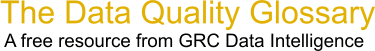Choose Index below for a list of all words and phrases defined in this glossary.

# Beta Risk

index | Index

## Beta Risk - definition(s)

Beta Risk - Beta risk is defined as the risk of accepting the null hypothesis when, in fact, it is false.

Consumer Risk or Type II Risk.

Beta risk is defined as the risk of accepting the null hypothesis when, in fact, the alternate hypothesis is true. In other words, stating no difference exists when there is an actual difference. A statistical test should be capable of detecting differences that are important to you, and beta risk is the probability (such as 0.10 or 10%) that it will not. Beta risk is determined by an organization or individual and is based on the nature of the decision being made. Beta risk depends on the magnitude of the difference between sample means and is managed by increasing test sample size. In general, a beta risk of 10% is considered acceptable in decision making.

The value (1-beta) is known as the "power" of a statistical test. The power is defined as the probability of rejecting the null hypothesis, given that the null hypothesis is indeed false.

-----------------

Actually, the term "risk" really means probability or chance of making an incorrect decision. The actual risks of making a wrong decision are unique to the decision being made and may be realized only if a wrong decision is made. For example, the probability of a false negative (type II error) on an a test for aids in a patient might be calculated as 0.001. The risk of concluding that a person does not have aids when in fact they do is quite a different concept - the "risk" is propagation of a deadly disease.

[Category=Data Quality ]

Source: iSixSigma, 01 January 2011 09:41:28, http://www.isixsigma.com/index.php?option=com_glossaryData Quality Glossary.  A free resource from GRC Data Intelligence. For comments, questions or feedback: dqglossary@grcdi.nl